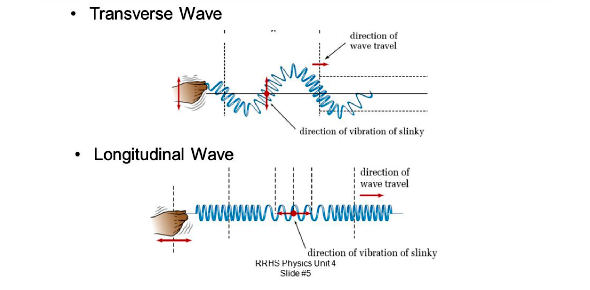# A Quick Mechanical Waves Quiz!

10 Questions | Attempts: 169549
ShareSettingsAre you familiar with mechanical waves and their concepts? A quick Mechanical Waves quiz will help you test your knowledge. A mechanical wave is the type of wave that needs a medium to be transmitted, as waves of this type do not travel through a vacuum. The quiz below is designed to test your basic understanding of mechanical waves. It will take less than a minute and is a true or false question. Give it a shot and see if you need more reading.

• 1.
Waves are a transfer of momentum and energy via a medium.
• A.

True

• B.

False

• 2.
Mechanical waves don't require a medium.
• A.

True

• B.

False

• 3.
Transverse Wave:
• A.

Is one in which the medium is displaced perpendicular to the wave in the direction of wave propagation, such as waves on a string

• B.

Is one in which the medium is displaced parallel to the wave in the direction of wave propagation, such as waves on a string

• C.

Is one in which the medium is displaced perpendicular to the wave in the direction of wave propagation, such as a sound wave in air

• D.

Is one which the medium is displaced perpendicular to the wave in direction of wave propagation, such as sound wave in air.

• 4.
Longitudinal Wave:
• A.

Is one in which the medium is displaced perpendicular to the wave in the direction of wave propagation, such as waves on a string

• B.

Is one in which the medium is displaced parallel to the wave in the direction of wave propagation, such as waves on a string

• C.

Is one in which the medium is displaced perpendicular to the wave in the direction of wave propagation, such as a sound wave in air

• D.

Is one in which the medium is displaced parallel to the wave in the direction of wave propagation, such as a sound wave in the air

• 5.
Highest point:
• 6.
What kind of waves vibrates our eardrums so we are able to listen?
• A.

• B.

Sound waves

• C.

Light waves

• D.

Microwaves

• 7.
Mechanical Waves can travel through a vacuum.
• A.

Yes

• B.

No

• 8.
Which one of the following is a sound wave?
• A.

Longitudinal and mechanical.

• B.

Transverse and mechanical.

• C.

Transverse and electromagnetic.

• D.

None of the above.

• 9.
A transverse wave can be created in a Slinky by moving it.
• A.

In a circular motion

• B.

Up and down

• C.

Side to side

• D.

Together and apart

• 10.
Amplitude reflects the amount of _______ in a wave.
• A.

Energy

• B.

Speed

• C.

Rarefaction

• D.

Compression

## Related TopicsBack to top
×

Wait!
Here's an interesting quiz for you.